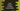# Java program to check if a number is a spy number or not## Java program to check if a number is spy number or not:

In this post, we will learn how to check if a number is a spy number or not. It will take one number as input from the user and print one message if it is a spy number or not.

A number is called a spy number if the product of the digits of the number is equal to the sum of the digits of that number.

For example, 123 is a spy number because the sum of its digits 1 + 2 + 3 is 6 which is equal to the product of its digits 1 * 2 * 3.

Before we start to write the program, let’s check the algorithm to use.

### Algorithm to check if a number is spy number or not:

We will use the below algorithm:

• Take the number as input from the user.
• Initialize two variables to hold the sum and product of the digits of the number. Initialize the sum variable as 0 and product variable as 1.
• Run one loop that will run until the value of the number is greater than 0.

• On each iteration, get the last digit of the number. Add it to the sum variable and assign this value as the value of the sum variable. Similarly, multiply it with the product variable and assign this value as the value of the product variable.
• Remove the last digit from the number.
• At the end, check if the value of sum is equal to product or not. If yes, it is a spy number.

### Java program:

Let’s take a look at the program:

``````import java.util.Scanner;

public class Main {

public static void main(String[] args) {
int n, lastDigit;
int sum = 0, product = 1;
Scanner sc = new Scanner(System.in);

System.out.println("Enter a number: ");
n = sc.nextInt();

while (n > 0) {
lastDigit = n % 10;
sum += lastDigit;
product *= lastDigit;

n /= 10;
}

if (sum == product) {
System.out.println("It is a spy number");
} else {
System.out.println("It is not a spy number");
}

}
}``````

Here,

• n is an integer to hold the user input number. lastDigit is to hold the last digit of the number.
• sum is to hold the sum of digits of the number. It is initialized as 0. product is to hold the product of digits of the number and it is initialized as 1.
• It reads the user input number by using a Scanner object.
• The while loop runs until the value of n is more than 0. On each iteration, it gets the last digit of the number and adds it to sum and multiply it to product. It also removes the last digit of the number by dividing the number by 10.
• The last if-else block checks if the value of sum is equal to product or not. Based on that, it prints one message.

If you run this program, it will print output as like below:

``````Enter a number:
124
It is not a spy number

Enter a number:
123
It is a spy number``````

### Java program to check for a spy number by using a separate method:

We can also use a separate method to check for a spy number. This method will be called from the main method and it will return one boolean value.

Using a separate method has many advantages like we can call this method from different parts of a program.

Below is the complete program:

``````import java.util.Scanner;

public class Main {

public static boolean isSpy(int n) {
int lastDigit;
int sum = 0, product = 1;

while (n > 0) {
lastDigit = n % 10;
sum += lastDigit;
product *= lastDigit;

n /= 10;
}
return sum == product;
}

public static void main(String[] args) {
int n;
Scanner sc = new Scanner(System.in);

System.out.println("Enter a number: ");
n = sc.nextInt();

if (isSpy(n)) {
System.out.println("It is a spy number");
} else {
System.out.println("It is not a spy number");
}

}
}``````

Here,

• We have created another method isSpy to check for a spy number. This method takes one integer as the parameter and returns one boolean value.
• This method is called from main and based on its return value, we are printing one message.
• This method is a static method, so we can call it from any other files without creating a new object.

It will give similar result.

``````Enter a number:
123
It is a spy number``````

### Find all spy numbers between 1 to 1000:

Let’s write a program that finds and prints all spy numbers between 1 to 1000. We will use a loop that will run from 1 to 1000 and use the above method to check in each iteration if a number is spy or not.

You can change the range of the loop to any other value as well. You can also take the range values as input from the user.

``````public class Main {
public static boolean isSpy(int n) {
int lastDigit;
int sum = 0, product = 1;

while (n > 0) {
lastDigit = n % 10;
sum += lastDigit;
product *= lastDigit;

n /= 10;
}
return sum == product;
}

public static void main(String[] args) {
for (int i = 1; i <= 1000; i++) {
if (isSpy(i)) {
System.out.print(i + " ");
}
}
}
}``````

Here,

• We are using a for loop that runs from 1 to 1000 for i
• For each value of i, it uses isSpy method to check if that value is a spy number or not.
• If yes, it prints that value.

If you run this program, it will print:

``1 2 3 4 5 6 7 8 9 22 123 132 213 231 312 321 ``